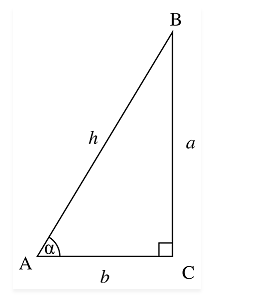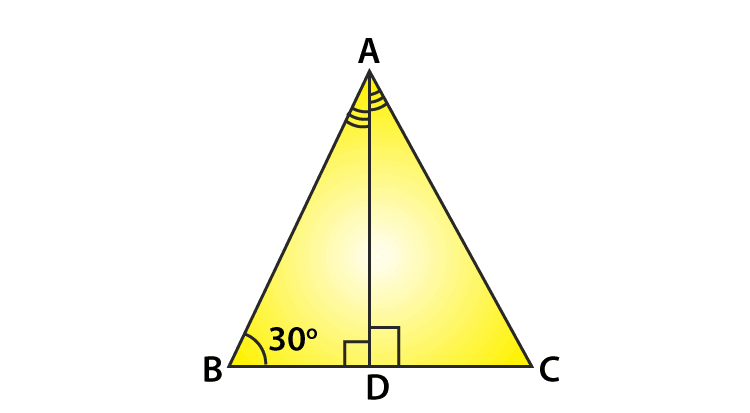# Sec 30

The value of Sec 30 degrees is equal to 2/√3. In trigonometry, you may have learned about three main primary functions, such as sine, cosine and tangent along with them the other three trigonometric functions, such as secant, cotangent and cosecant. Here, you will find the value of sec 30 degrees along with the other secant degree values.

Secant function is the transpose of the cosine function. Therefore, to find the value of sec 30, we have to find the value of cos 30. We take the reference of a right-angle triangle to get the values of trigonometric functions or ratios such as sin, cos, tan, sec, cot, csc.

value of sec 30° = 2/√3

As we know, Cosine function defines a relation between the adjacent side and the hypotenuse of a right-angled triangle with respect to the angle, formed between the adjacent side and the hypotenuse. Therefore, we can say the secant function defines a relation between the hypotenuse and adjacent side of the right-angled triangle with respect to the angle, formed between the adjacent side and the hypotenuse. Hence, the sec 30° value will be the ratio of the hypotenuse and adjacent side.

We will derive the value of sec 30 degrees with the help of cos function. Also, the values of other degrees such as 0°, 45°, 60°, 90° which are generally used in trigonometry equations.

## What is Sec 30 Value?

We know that, in a right-angled triangle, the secant of ∠α is a ratio of the length of the hypotenuse and the adjacent side to the angle, where ∠α is the angle formed between the adjacent side and the hypotenuse.= Hypotenuse/Base

sec ∠α = h/b

The value of sec 30 degrees is 2√3.

The value of sec 30 in radians is π/180°.

Let us derive the value of secant 30 in the next section.

### Derivation of Sec 30 DegreesSince, we know,

sec A = 1/cos A

From the above figure, in triangle ABD,

sec 300 = 1/cos 300

Since the value of cos 300 = √3/2

Hence, the value of sec 300 = 2/√3

In the same way, we can write the other values sec ratios, such as,

sec 00 = 1/cos 0= 1/1 = 1

sec 450 = 1/cos 450 = 1/1/√2 = √2

sec 600 = 1/cos 600 = 1/1/2= 2

sec 900 = l/cos 900 = 1/0= ∞

Given below is the trigonometry table for the trigonometric ratios covering the values of degrees 00, 300, 450, 600, 900.

 00 300 450 600 900 Sin 0 1/2 1/√2 √3/2 1 Cos 1 √3/2 1/√2 1/2 0 Tan 0 1/√3 1 √3 ∞ Sec 1 2/√3 √2 2 ∞ Csc ∞ 2 √2 2/√3 1 Cot ∞ √3 1 1/√3 0

## Sec 30 Example Problem

Example: Compute 2 sec 300 + 2 cos 600.

Solution:

Given,

2 sec 300 + 2 cos 600

We know, sec 300 = 2/√3 and cos 600 = 1/2

Therefore,

2 sec 300 + 2 cos 600 = 2 × 2/√3 + 2 × ½

= 4/√3 + 1

2 sec 300 + 2 cos 600= 4/√3 + 1.

## Frequently Asked Questions – FAQs

Q1

### What is the exact value of sin 30?

The exact value of sec 30° is 2/√3
Q2

### What is the formula for sec 30°?

The formula to find sec of an angle is the ratio of hypotenuse and adjacent side of the angle.
Q3

### What is the value of cos 45°?

The value of cos 45 degrees is 1/√2.
Q4

### Is the value of sec 30 and cos 30, same?

The value of sec 30 and cos 30 is different. Sec 30 = 2/√3 and Cos 30 = √3/2
Test your Knowledge on Sec 30